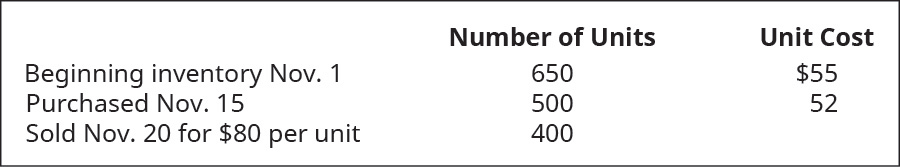# Calculate the cost of goods sold dollar value for B74 Company for the sale on November 20, considering the following transactions under three different cost allocation methods and using perpetual inventory updating. Provide calculations for (a) first-in, first-out (FIFO); (b) last-in, first-out (LIFO); and (c) weighted average (AVG).FindFindarrow_forward

### Principles of Accounting Volume 1

19th Edition
OpenStax
Publisher: OpenStax College
ISBN: 9781947172685

#### Solutions

Chapter
SectionFindFindarrow_forward

### Principles of Accounting Volume 1

19th Edition
OpenStax
Publisher: OpenStax College
ISBN: 9781947172685
Chapter 10, Problem 4PB
Textbook Problem
1 views

## Calculate the cost of goods sold dollar value for B74 Company for the sale on November 20, considering the following transactions under three different cost allocation methods and using perpetual inventory updating. Provide calculations for (a) first-in, first-out (FIFO); (b) last-in, first-out (LIFO); and (c) weighted average (AVG).To determine

(a)

Concept introduction:

Cost of goods sold: It is the cost which is also known as cost of goods manufactured, calculates the total value of goods produced during the period and is ready for sale. In other word total amount of expense incurred to turn raw material inventory into finished goods.

To calculate:

Cost of goods sold as per FIFO.

### Explanation of Solution

To calculate the cost of goods sold company multiply the cost per unit to the quantity sold...

To determine

(b)

Concept introduction:

Cost of goods sold: It is the cost which is also known as cost of goods manufactured, calculates the total value of goods produced during the period and is ready for sale. In other word total amount of expense incurred to turn raw material inventory into finished goods.

To calculate:

Cost of goods sold as per LIFO.

To determine

(c)

Concept introduction:

Cost of goods sold: It is the cost which is also known as cost of goods manufactured, calculates the total value of goods produced during the period and is ready for sale. In other word total amount of expense incurred to turn raw material inventory into finished goods.

To calculate:

Cost of goods sold as per weighted average.

### Still sussing out bartleby?

Check out a sample textbook solution.

See a sample solution

#### The Solution to Your Study Problems

Bartleby provides explanations to thousands of textbook problems written by our experts, many with advanced degrees!

Get Started

Find more solutions based on key concepts
Which fluctuate morelong-term or short-term interest rates? Why?

Fundamentals of Financial Management (MindTap Course List)

What is human capital management (HCM)?

Pkg Acc Infor Systems MS VISIO CD

Discuss the following statement: A bonds yield to maturity is the bonds promised rate of return, which equals i...

Fundamentals of Financial Management, Concise Edition (with Thomson ONE - Business School Edition, 1 term (6 months) Printed Access Card) (MindTap Course List)

Why must researchers document their sources meticuousIy?

Essentials of Business Communication (MindTap Course List)

Understand the sales environment

MKTG 12:STUDENT ED.-TEXT